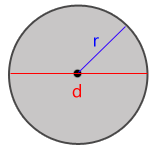# Calculate the area of a circle

Use this calculation to calculate the area of a circle. You can use numerous different inputs and choose to submit the radius, the diameter or the circumference of the circle. In the result you will get all unknown variables presented.#### What is broken?

Is there something wrong with the calculation Calculate the area of a circle? Is it a bug or has it gone completely offline? Please, let us know what is wrong! If the calculation did not give you the result you expected, please write which values you used and what you expected the calculation to do.

#### Bugs & Fixes

This calculation was last reviewed or fixed: 2011/09/29

Bug / Fix When Name Comment